# Multiple Choice Questions Elasticity

In this page we have Multiple Choice Questions of Elasticity for JEE Main/Advanced and NEET . Hope you like them and do not forget to like , social share and comment at the end of the page.

## Multiple Choice questions

Question 1
A wire whose cross-section area is A1 is stretched by L1 by a certain weight. How far will a wire of same material and same length and cross-section area A2 stretch if same weight is applied to it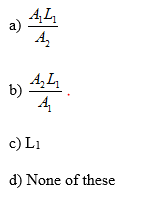Question 2
A wire is stretched by x mm when a load F is hanged on it.If the same wire goes over a pulley and two weight F each are hung at the two end’s the what will be the elongation in the wire
(a) x
(b) 2x
(c) x/2
(d) 0

(A) One end of a uniform wire of length L and μ mass per unit length is attached rigidly to a point in a ceiling. A Mass M is suspended from its lower end. Area of cross-section of the wire is A.
Question 3
Find the stress at a distance x from the ceiling point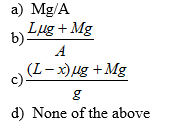Question 4
Find the elongation of the wire. If Young Modulus of wire is Y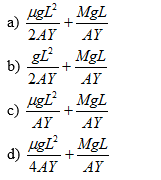## Multiple Choice questions

Question 5
A block of gelatin is 60 mm by 60 mm by 20 mm when unstressed. A force of .245 N is applied tangentially to the upper surface causing a 5mm displacement relative to the lower surface. The block is placed such that 60X60 comes on the lower and upper surface. Find the shearing stress, shearing strain and shear modulus
(a) (68.1 N/m2,.25,272.4 N/m2)
(b) (68 N/m2,.25,272 N/m2)
(c) (67 N/m2,.26,270.4 N/m2)
(d) (68.5 N/m2,.27,272.4 N/m2)

## Paragraph Based Questions

(B) A wire of radius r is stretched without sag and tension between two point separated by distance 2L.A weight is hanged at the middle of the wire which displaced the point by a distance d. Young modulus of elasticity is Y.

Question 6
Find the tension in the wire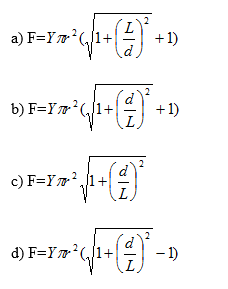Question 7
If in previous question d<<<<L, what will be the value of Tension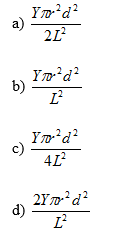## Multiple Choice Questions

Question 8
A spring is stretched by applying a load to its free end. The strain produced in the spring is
(a) volumetric.
(b) shear.
(c) longitudinal and shear.
(d) longitudinal.

Question 9
A mild steel wire of length 2L and cross-sectional area A is stretched, well within elastic limit, horizontally between two pillar. A mass m is suspended from the mid point of the wire.Strain in the wire is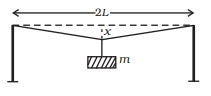(a) $\frac {x}{L}$
(b) $\frac {x^2}{2L^2}$
(c) $\frac {x^2}{2L}$
(d) $\frac {x^2}{L}$

Question 10
The stress versus strain graphs for wires of two materials A and B are as shown in the figure. If $Y_A$ and $Y_B$ are the Young 's modulii of the materials, then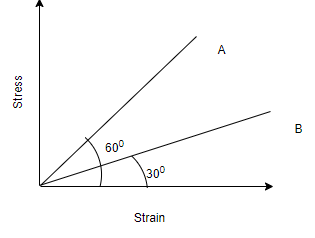(a) $Y_A = 2 Y_B$
(b) $Y_B= 3 Y_A$
(c) $Y_A= 3 Y_B$
(c) $Y_A= 2 Y_B$

Question 11
The adjacent graph shows the extension ($\Delta L$) of a wire of length 1m suspended from the top of a roof at one end with a load W connected to the other end. If the cross sectional area of the wire is $10^{-6} \ m^2$, calculate the young?s modulus of the material of the wire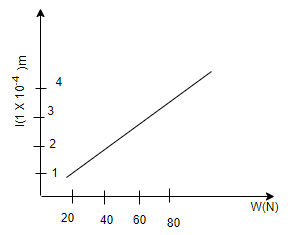(a) $2 \times 10^{11} \ (N / m^2)$
(b) $2 \times 10^{-11} \ (N / m^2)$
(c) $2 \times 10^{-12} \ (N / m^2)$
(d) $2 \times 10^{-12} \ (N / m^2)$

Question 12
A wire is suspended from the ceiling and stretched under the action of a weight F suspended from its other end. The force exerted by the ceiling on it is equal and opposite to the weight.
(a) Tensile stress at any cross section A of the wire is F/A.
(b) Tensile stress at any cross section is zero.
(c) Tensile stress at any cross section A of the wire is 2F/A.
(d) Tension at any cross section A of the wire is F

Question 13
One end of a uniform wire of length L and of weight W is attached rigidly to a point in the roof and a weight W1 is suspended from its lower end. If S is the area of cross-section of the wire, the stress in the wire at a height 3L/4 from its lower end is
(a) $\frac {W_1}{S}$
(b) $\frac {W_1 + W/4}{S}$
(c) $\frac {W_1 + 3W/4}{S}$
(d) $\frac {W_1 + W}{S}$

Question 14
For an ideal liquid
(a) the bulk modulus is infinite.
(b) the bulk modulus is zero.
(c) the shear modulus is infinite.
(d) the shear modulus is zero.